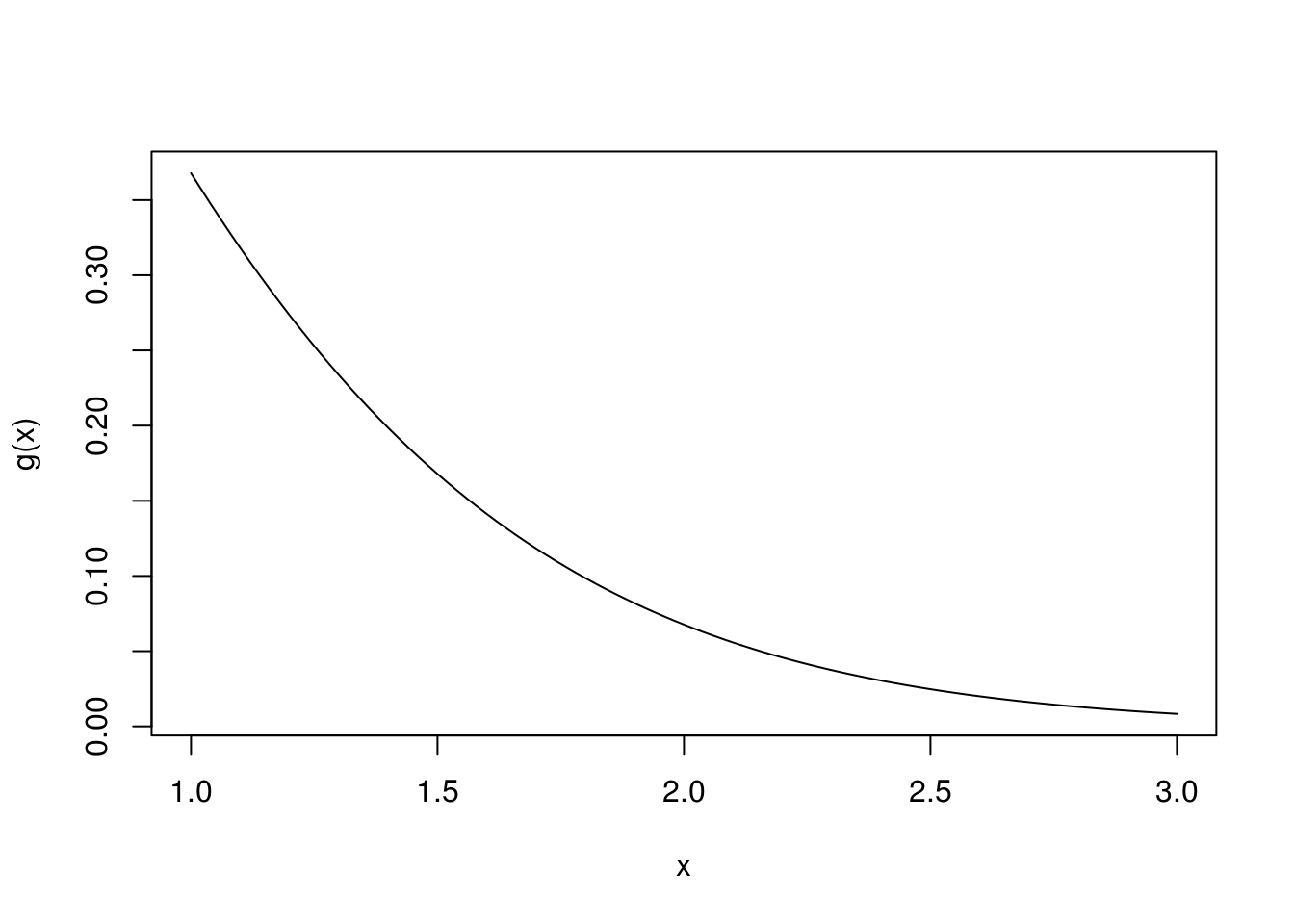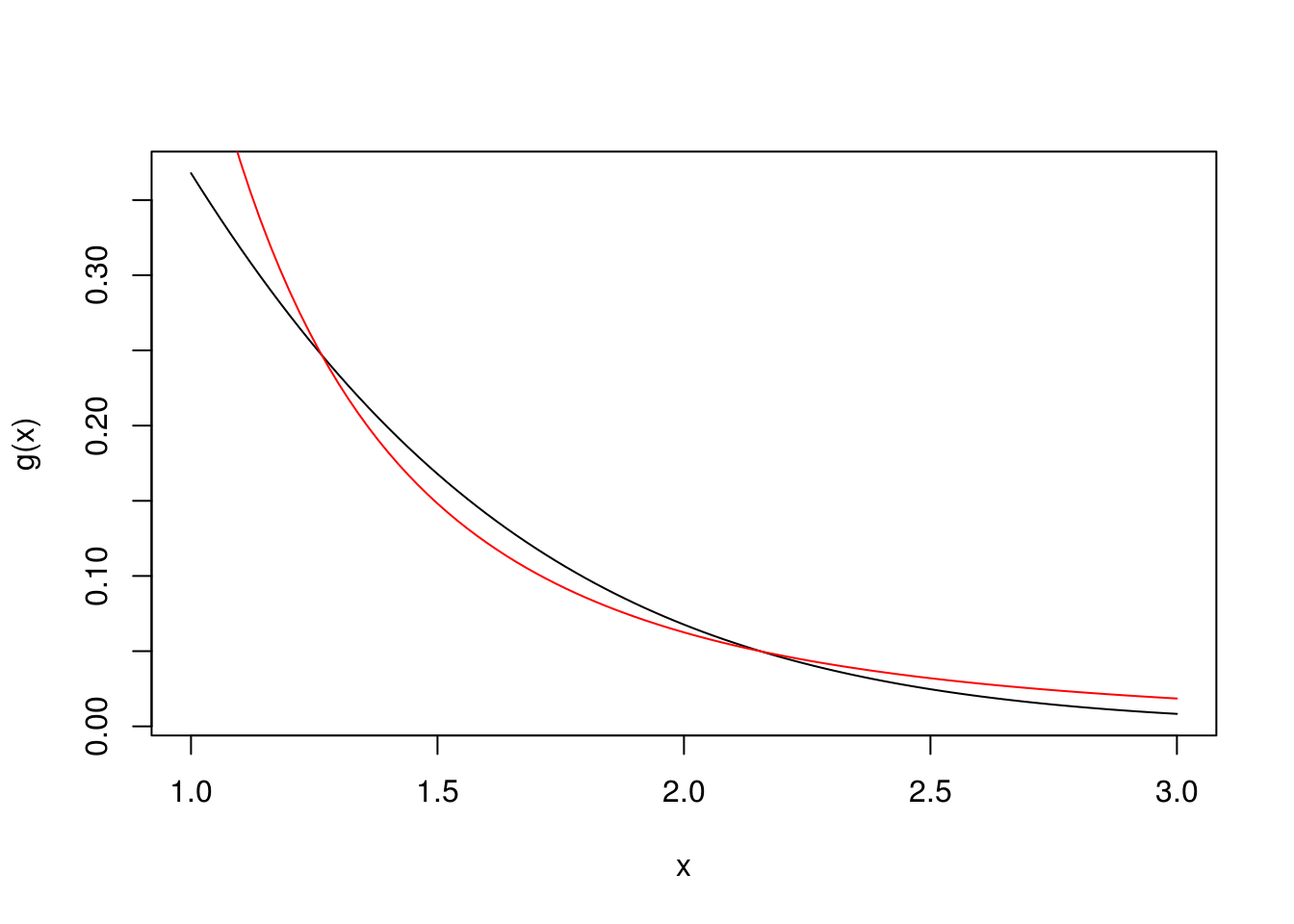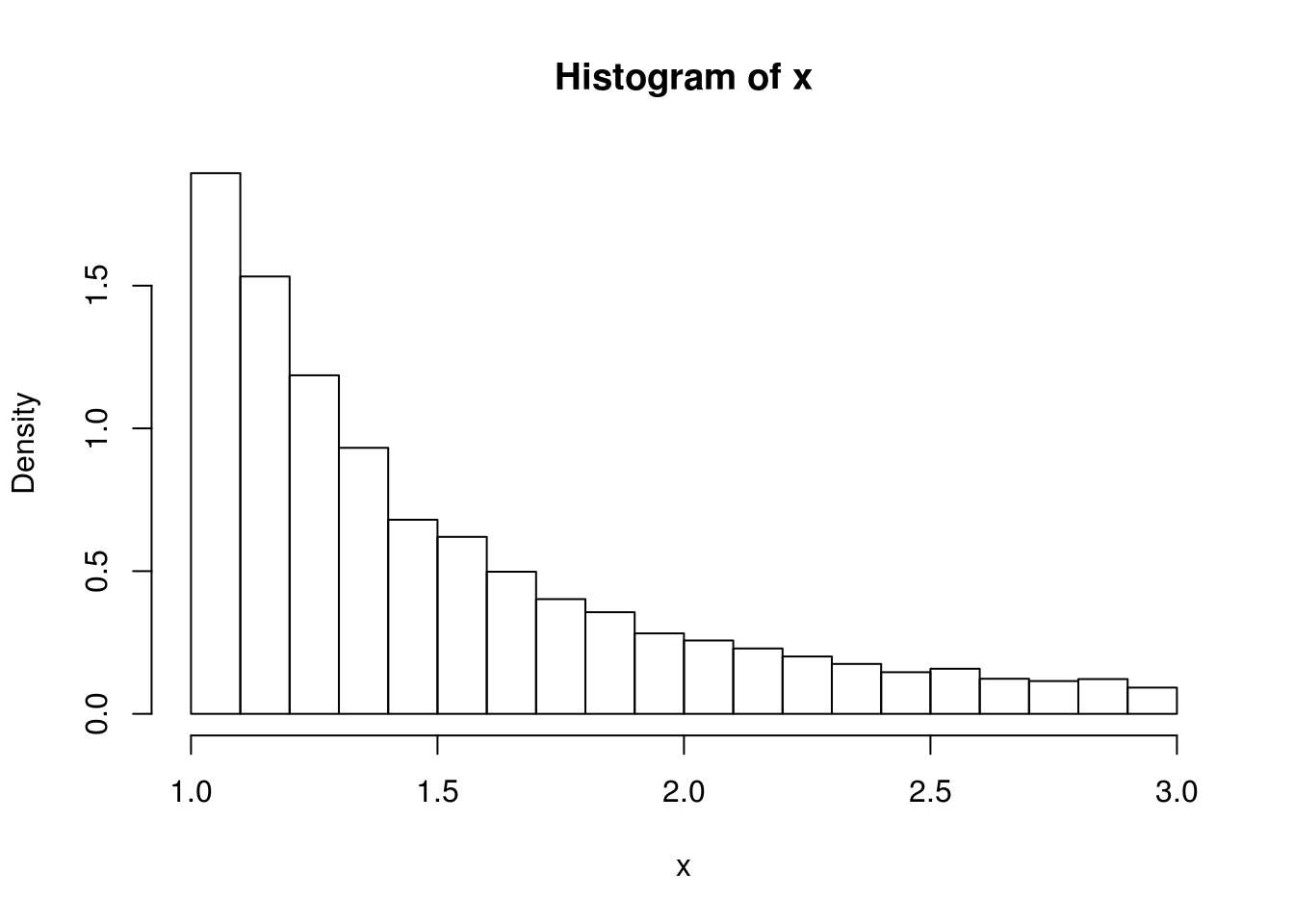# Details

Consider a function $$g$$ on the real line. Suppose we want to integrate $$g$$ over $$[a,b]$$:

$\int_a^b g(x) \ dx.$

Suppose that we have a density $$p$$ for which $$p(x)>0$$ for all $$x \in [a,b]$$. Remember that the idea of Monte Carlo integration is to write the quantity of interest as an expectation, as follows:

\begin{align*} \int_a^b g(x) \ dx & = \int_a^b \frac{g(x)}{p(x)} \ p(x) \ dx \\ & = \int \frac{g(x)}{p(x)} \ \text{I}\{ a \le x \le b \} \ p(x) \ dx \\ & = \text{E} \bigg( \frac{g(X)}{p(X)} \ \text{I}\{ a \le X \le b \} \bigg) \\ & = \text{E} ( h (X) ) \\ \end{align*}

Now do the usual steps in Monte Carlo integration (e.g., sampling many $$X$$’s, apply the function $$h$$ to each $$X$$, find the arithmetic mean, and assess Monte Carlo uncertainity).

Does it matter which density $$p$$ we use? In theory, no. In practice, we want a density $$p$$ having the following desirable properties:

1. The density $$p$$ is easy to evaluate,
2. The distribution corresponding to the density $$p$$ is easy to sample, and
3. The variance of $$\frac{g(X)}{p(X)} \ \text{I}\{ a \le X \le b \}$$ is small. That this, $$p$$ matches the shape of $$g$$ well on $$x \in [a,b]$$.

# Example

Evaluate: $\int_1^3 \frac{1}{ x \ \Gamma(x) \ e^x } \ dx.$

g <- function(x) 1 / ( x * gamma(x) * exp(x) )
curve(g(x),1,3)Consider a density $$p$$ of the following form: $$p(x) = \frac{c}{2x^3}$$ for $$x \in [1,3]$$.

curve(g(x),1,3)
curve(1/(2*x^3),1,3,add=TRUE,col="red")Notice that $$p$$ matches the shape of $$h$$ well (“Desirable Properties 3”).

Since $$p$$ is a density, we can find $$c$$ by solving the following:

$1 = \int_1^3 \frac{c}{2x^3} dx \ \implies c = 9/2.$

So, $$p(x) = \frac{9}{4x^3}$$ for $$x \in [1,3]$$.

p <- function(x) 9 / (4*x^3)

Notice that $$p$$ is easy to evaluate (“Desirable Properties 1”).

## Application of the Probability Integral Transform

How can we sample from the distribution having density $$p$$? Use the probability intergral transform. First, find $$F$$, the distribution function associated with the density $$p$$:

$F(x) = \int_1^x \frac{9}{4t^3} \ dt = \frac{9}{8}\bigg( 1 - \frac{1}{x^2} \bigg) \ \ \text{for} \ x \in [1,3].$

Now find $$F^{-1}$$, the inverse of the c.d.f. $$F$$:

$F^{-1}(y) = \bigg( 1 - \frac{8}{9} y \bigg)^{-\frac{1}{2}} \ \ \text{for} \ y \in [0,1].$

Finally, get samples from the distribution having density $$p$$ by apply the inverse c.d.f. to draws from the uniform distribution on the unit interval.

Finv <- function(y) 1/sqrt(1-8*y/9)
B <- 10000
x <- Finv(runif(B))

Notice that it is easy and quick to obtain samples from the distribution (“Desirable Properties 2”).

Check ourselves by noticing they seems to have a histogram with the right shape.

hist(x,freq=FALSE)## Putting It All Together

Now apply standard Monte Carlo integration techniques to $$h$$.

h <- function(x) g(x)/p(x) * (x>=1) * (x<=3)
est <- mean(h(x))
cat(sprintf("Monte Carlo estimate is %7.5f.\n",est))
## Monte Carlo estimate is 0.21303.
ci <- est + c(-1,1)*qnorm(0.975)*sd(h(x))/sqrt(B)
cat(sprintf("The 95%% confidence interval is (%7.5f,%7.5f).\n",ci,ci))
## The 95% confidence interval is (0.21234,0.21372).

## The Choice of $$p$$ Matters

Instead of this $$p(x) = \frac{9}{4x^3}$$ for $$x \in [1,3]$$, what if we had just used a uniform distribution $$[1,3]$$, i.e., $$p(x) = 1/2$$ for $$x \in [1,3]$$?

x <- runif(B,1,3)
h <- function(x) g(x)/(1/2) * (x>=1) * (x<=3)
est <- mean(h(x))
cat(sprintf("Monte Carlo estimate is %7.5f.\n",est))
## Monte Carlo estimate is 0.21336.
ci <- est + c(-1,1)*qnorm(0.975)*sd(h(x))/sqrt(B)
cat(sprintf("The 95%% confidence interval is (%7.5f,%7.5f).\n",ci,ci))
## The 95% confidence interval is (0.20947,0.21725).

What do you notice is difference about the two solutions?